Study Guide

# Basic Geometry - Angles

## Angles

Not to be confused with angels, angles are the pointed corners of shapes. Angles can be named three different ways.

This angle could be named any of these ways:

•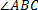(counterclockwise)
•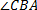(clockwise)
•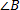(just the vertex)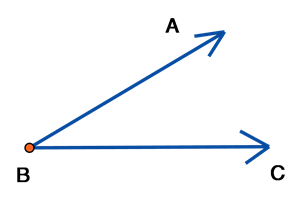Look Out: when naming an angle using three letters, the vertex must always be the middle letter!

### Types of Angles

 Name Description Example Acute Angle An angle less that 90°. Lame joke: this angle is so small and a-cute!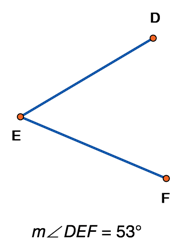Obtuse Angle An angle greater than 90°, but less than 180°.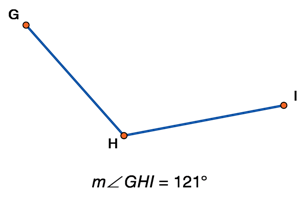Right Angle An angle that is exactly 90°. This is commonly denoted with a small box in the corner.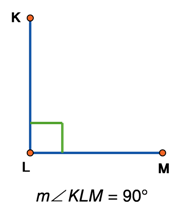Straight Angle A 180° angle. It's - you guessed it - a straight line.Supplementary Angles and Linear Pairs Angles that add up to 180°.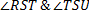are supplementary angles.If the two angles are adjacent (next to each other) and add up to 180°, they are a linear pair.are also a linear pair.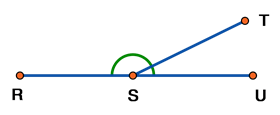Complementary Angles Angles that add up to 90°.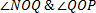are complementary.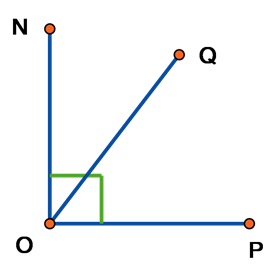Vertical Angles When two lines intersect, opposite angles are called "vertical angles". Vertical angles are congruent.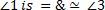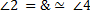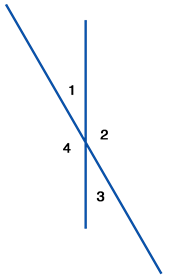See the squiggly line over the equal sign above? This symbol means congruent. Oh, and btw, congruent angles have the same measurement.